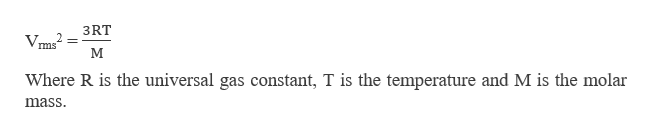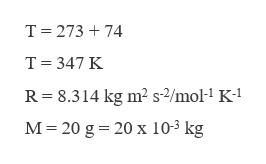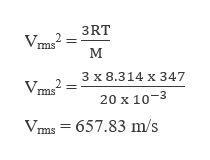# Two vessels are labeled A and B. Vessel A contains NH3 gas at 74°C, and vessel B contains Ne gas at the same temperature. If the average kinetic energy of NH3 is 7.1 ×10−21 J/molecule at 70°C, calculate the root-mean-square speed of Ne atoms in m/s.

Question
21 views

Two vessels are labeled A and B. Vessel A contains NH3 gas at 74°C, and vessel B contains Ne gas at the same temperature. If the average kinetic energy of NH3 is 7.1 ×10−21 J/molecule at 70°C, calculate the root-mean-square speed of Ne atoms in m/s.

check_circle

Step 1

The root mean square speed can be calculated as,help_outlineImage Transcriptionclose3RT ms Where R is the universal gas constant, T is the temperature and M is the molar mass fullscreen
Step 2

The temperature is given as 74 oC, converting it in Kelvin,help_outlineImage TranscriptioncloseT 273 74 T 347 K R 8.314 kg m2 s-2/mol-1 K-1 M 20 g 20 x 10-3 kg fullscreen
Step 3

Substituting the...help_outlineImage Transcriptionclose3RT ms м 3 x 8.314 x 347 Vms2 20 x 10-3 ms = 657.83 m/s fullscreen

### Want to see the full answer?

See Solution

#### Want to see this answer and more?

Solutions are written by subject experts who are available 24/7. Questions are typically answered within 1 hour.*

See Solution
*Response times may vary by subject and question.
Tagged in

### Chemistry